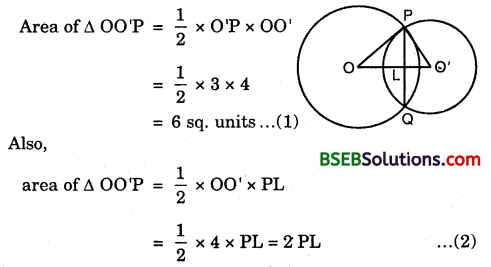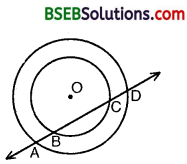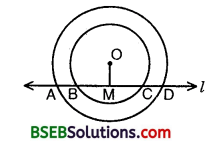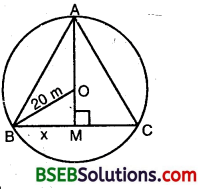# HSSlive: Plus One & Plus Two Notes & Solutions for Kerala State Board

## BSEB Class 9 Maths Chapter 10 Circles Ex 10.4 Textbook Solutions PDF: Download Bihar Board STD 9th Maths Chapter 10 Circles Ex 10.4 Book AnswersBSEB Class 9 Maths Chapter 10 Circles Ex 10.4 Textbook Solutions PDF: Download Bihar Board STD 9th Maths Chapter 10 Circles Ex 10.4 Book Answers

BSEB Class 9th Maths Chapter 10 Circles Ex 10.4 Textbooks Solutions and answers for students are now available in pdf format. Bihar Board Class 9th Maths Chapter 10 Circles Ex 10.4 Book answers and solutions are one of the most important study materials for any student. The Bihar Board Class 9th Maths Chapter 10 Circles Ex 10.4 books are published by the Bihar Board Publishers. These Bihar Board Class 9th Maths Chapter 10 Circles Ex 10.4 textbooks are prepared by a group of expert faculty members. Students can download these BSEB STD 9th Maths Chapter 10 Circles Ex 10.4 book solutions pdf online from this page.

## Bihar Board Class 9th Maths Chapter 10 Circles Ex 10.4 Books Solutions

 Board BSEB Materials Textbook Solutions/Guide Format DOC/PDF Class 9th Subject Maths Chapter 10 Circles Ex 10.4 Chapters All Provider Hsslive

## How to download Bihar Board Class 9th Maths Chapter 10 Circles Ex 10.4 Textbook Solutions Answers PDF Online?

2. Click on the Bihar Board Class 9th Maths Chapter 10 Circles Ex 10.4 Answers.
3. Look for your Bihar Board STD 9th Maths Chapter 10 Circles Ex 10.4 Textbooks PDF.
4. Now download or read the Bihar Board Class 9th Maths Chapter 10 Circles Ex 10.4 Textbook Solutions for PDF Free.

Find below the list of all BSEB Class 9th Maths Chapter 10 Circles Ex 10.4 Textbook Solutions for PDF’s for you to download and prepare for the upcoming exams:

## BSEB Bihar Board Class 9th Maths Solutions Chapter 10 Circles Ex 10.4

Question 1.
Two circles of radii 5 cm and 3 cm intersect at two points and the distance between their centres is 4 cm. Find the length of the common chord.
Solution:
Let O and O’ be the centres of the circles of radii 5 cm and 3 cm respectively and let PQ be them common chord.
We have OP = 5 cm, O’P = 3 cm and OO’ = 4 cm.
Since OP² = PO’² + O’O² [∵ 5² = 3² + 4²]
⇒ OOP is a right ∠d ∆, right angled as O’.From (1) and (2), we have
2 x PL = 6 ⇒ PL = 3
We know that when two circles intersect at two points, then their centre lie on the perpendicular bisector of the common chord i.e., 00′ is the perpendicular bisector of AB.
∴ PQ = 2 x PL = (2 x 3) cm = 6 cm.

Question 2.
If two equal chords of a circle intersect within the circle, prove that the segments of one chord are equal to corresponding segments of the other chord.
Solution:
Given : AB and CD are chords of a circle with centre O. AB and CD intersect at P and AB = CD.
To prove : (i) AP = PD (ii) PB = CP.Construction : Draw OM ⊥ AB, ON ⊥ CD.
Join OP.
AM = MB = 12[Perpendicular from centre bisects the chord]
CN = ND = 12 CD [Perpendicular from centre bisects the chord]
AM = ND and MB = CN s …(1) [∵ AB = CD (given)]
In As OMP and ONP, we have
OM = ON
[Equal chords of a circle are equidistant from the centre]
∠OMP = ∠ONP [∵ Each = 90°]
OP = OP [Common]
By RHS criterion of congruence,
∆ OMP = ∆ ONP
⇒ MP = PN … (2) [C.P.C.T.]
Adding (1) and (2), we have
AM + MP = ND + PN ⇒ AP = PD
Subtracting (2) from (1), we have
MP – MP = CN – PN
PB = CP
Hence, (i) AP = PD and (ii) PB = CP.

Question 3.
If two equal chords of a circle intersect within ‘ the circle, prove that the line joining the point of intersection to the centre makes equal angles with the chords.
Given : AB and CD are chords of a circle with centre O. AB and CD intersect at P and AB = CD.
To prove : ∠OPE = ∠OPF.Construction : Draw OE ⊥ AB and OF ⊥ CD, Join OP.
Solution:
In ∆s OEP and OFP, we have
∠OEP = ∠OFP [∵ Each = 90°]
OP = OP
OE = OF [Equal chords of a circle are equidistant from the centre]
∴ By RHS criterion of congruence
∆ OEP ≅ ∆ OFP
⇒ ∠OPE = ∠OPF

Question 4.
If a line intersects two concentric circles (circles with the same centre) with centre O at A, B, C and D, prove that AB = CD (see figure).Solution:
Let OM be perpendicular from O on line l. We know that the perpendicular from the centre of a circle to a chord, bisects the chord.
Since BC is a chord of the smaller circle and OM ⊥ BC.BM = CM … (1)
Again, AD is a chord of the larger circle and OM ⊥ AD.
∴ AM = DM … (2)
Subtracting (1) from (2), we get
AM – BM = DM – CM ⇒ AB = CD.

Question 5.
Three girls Reshma, Salma and Mandip are playing a game by standing on a circle of radius 5 m drawn in a park. Reshma throws a ball to Salma, Salma to Mandip, Mandip to Reshma. If the distance between Reshma and Salma and between Salma and Mandip is 6 m each, what is the distance between Reshma and Mandip?
Solution:
Let the three girls Reshma, Salma and Mandip are standing on the circle of radius 5 cm at points B, A and C respectively.We know that if AB and AC are two equal chords of a circle, then the centre of the circle lies on the bisector of ∠BAC.
Here, AB = AC = 6 cm. So, the bisector of ∠BAC passes through the centre O i.e., OA is the bisector of ∠BAC.
Since the internal bisector of an angle divides the opposite sides in the ratio of the sides containing the angle. Therefore, M divides BC in the ratio 6 : 6 = 1 : 1 i.e., M is the middle point of BC.
Now, M is the mid-point of BC ⇒ OM ± BC.
In right ∠d A ABM, we have
AB² = AM² + BM²
⇒ 36 = AM² + BM²
⇒ BM² = 36 – AM² … (1)
In the right A OBM, we have
OB² = OM² + BM²
⇒ 25 = (OA – AM)² + BM²
⇒ BM² = 25 – (OA – AM)²
⇒ BM² = 25 – (5 – AM)² … (2)
From (1) and (2), we get
36 – AM² = 25 – (5 – AM)²
⇒ 11 – AM² + (5 – AM)² = 0
⇒ 11 – AM² + 25 – 10AM + AM² = 0
⇒ 10AM = 36
⇒ AM = 3.6
Putting AM = 36 in (1), we get
BM² = 36 – (3.6)² = 36 – 12.96
⇒ BM = 36−12.96‾‾‾‾‾‾‾‾‾‾√ = 23.04‾‾‾‾‾√ = 4.8 cm
⇒ BC = 2BM = 2 x 4.8 = 9.6 cm
Hence, the distance between Reshma and Mandip = 9.6 cm.

Question 6.
A circular park of radius 20 m is situated in a colony. Three boys Ankur, Syed and David are sitting at equal distance on its boundary each having a toy telephone in his hands to talk each other. Find the length of the string of each phone.
Solution:
Let ABC is an equilateral triangle of side 2x metres.Clearly, BM = 𝐵𝐶2 = 2𝑥2 = x metres.
In right ∠d ∆ ABM,
AM² = AB² – BM²
= (2x)² – x² = 4x² – x² = 3x²
⇒ AM = 3‾√𝑥
Now, OM = AM – OA = (3‾√𝑥 – 20) m
In right ∠d ∆ OEM, we have
OB² = BM² + OM²
⇒ 20² = x² + (3‾√𝑥 – 20)²
⇒ 400 = x² + 3x² – 403‾√𝑥 + 400
⇒ 4x² – 403‾√𝑥 =0
⇒ 4x(x – 103‾√) = 0
Since x ≠ 0, ∴ x – 103‾√ = 0 ⇒ x = 103‾√
Now, BC = 2BM = 2x = 203‾√
Hence, the length of each string = 203‾√ m.

## Bihar Board Class 9th Maths Chapter 10 Circles Ex 10.4 Textbooks for Exam Preparations

Bihar Board Class 9th Maths Chapter 10 Circles Ex 10.4 Textbook Solutions can be of great help in your Bihar Board Class 9th Maths Chapter 10 Circles Ex 10.4 exam preparation. The BSEB STD 9th Maths Chapter 10 Circles Ex 10.4 Textbooks study material, used with the English medium textbooks, can help you complete the entire Class 9th Maths Chapter 10 Circles Ex 10.4 Books State Board syllabus with maximum efficiency.

## FAQs Regarding Bihar Board Class 9th Maths Chapter 10 Circles Ex 10.4 Textbook Solutions

#### Can we get a Bihar Board Book PDF for all Classes?

Yes you can get Bihar Board Text Book PDF for all classes using the links provided in the above article.

## Important Terms

Bihar Board Class 9th Maths Chapter 10 Circles Ex 10.4, BSEB Class 9th Maths Chapter 10 Circles Ex 10.4 Textbooks, Bihar Board Class 9th Maths Chapter 10 Circles Ex 10.4, Bihar Board Class 9th Maths Chapter 10 Circles Ex 10.4 Textbook solutions, BSEB Class 9th Maths Chapter 10 Circles Ex 10.4 Textbooks Solutions, Bihar Board STD 9th Maths Chapter 10 Circles Ex 10.4, BSEB STD 9th Maths Chapter 10 Circles Ex 10.4 Textbooks, Bihar Board STD 9th Maths Chapter 10 Circles Ex 10.4, Bihar Board STD 9th Maths Chapter 10 Circles Ex 10.4 Textbook solutions, BSEB STD 9th Maths Chapter 10 Circles Ex 10.4 Textbooks Solutions,
Share: